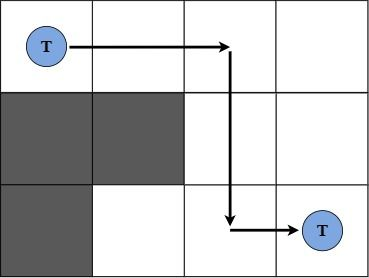# Birthday Present?!sayeef006Tough Duo, April 2022
Limits 1s, 256 MB

As everyone gifts graphs or strings to people on their birthdays, Oyshee’s friends decided to do something different and gave her a grid of size $N \times M$ on her birthday. Some of the cells of the grid are blocked but others are usable.

Overwhelmed with happiness, she decided to play a game on the grid. She placed a token on the cell $(1,1)$. Now she wants to move the token to the cell $(N,M)$.

To do this, she can build some directed bridges. If the token is currently at cell $A(x_1,y_1)$ and she builds a directed bridge from $A$ to $B(x_2,y_2)$, she can immediately move the token to cell $B$. But she can’t move the token from $B$ to $A$. Moving the token happens instantaneously. So, it doesn’t take any time. But building a bridge does.

She can build a directed bridge from $A$ to $B$ if one of the following two conditions is satisfied:

1. $x_1=x_2$ and $y_1, this takes her $(T+bridge\_length)$ seconds. Here, $bridge\_length$ = $(y_2-y_1)$.

2. $y_1=y_2$ and $x_1, this takes her $(T+bridge\_length)$ seconds. Here, $bridge\_length$ = $(x_2-x_1)$.

But she can not build a bridge of length more than $L$. She can not build a bridge to the outside of the grid. She also can not build a bridge over a blocked cell or place the token on a blocked cell. Two or more bridges may overlap each other. She can not move the token to other cells without bridges.

Now she’s wondering, what is the minimum time needed to move the token from cell $(1,1)$ to cell $(N,M)$?

## Input

The first line of input contains two integers $N$ and $M (2 \leq N,M \leq 3⋅10^3)$ - the size of the grid.

The next line contains two integers $L$ and $T (1 \leq L \leq 3⋅10^3, 1 \leq T \leq 10^9)$ - the maximum length of a bridge Oyshee can build, and the time added when building a bridge, respectively.

The following $N$ lines contain a string of $M$ characters each. Each character is either . or #. A . represents a usable cell and a # represents a blocked cell. Cell $(1,1)$ is guaranteed to be usable.

## Output

Print $-1$ if Oyshee can’t move the token to the cell $(N,M)$. Else, print the minimum possible time to do so.

## Samples

InputOutput
3 4
2 2
....
##..
#...

11Oyshee can move the token to cell $(3,4)$ in three steps:

1. Build a bridge from cell $(1,1)$ to $(1,3)$ and move the token to cell $(1,3)$, requiring time 2+2 = 4 seconds.

2. Build a bridge from cell $(1,3)$ to $(3,3)$ and move the token to cell $(3,3)$, requiring time 2+2 = 4 seconds.

3. Build a bridge from cell $(3,3)$ to $(3,4)$ and move the token to cell $(3,4)$, requiring time 2+1 = 3 seconds.

So the total time is (4+4+3) seconds = 11 seconds. This is the minimum time possible.

InputOutput
3 3
5 10
.##
.#.
#..

-1


Here, it is not possible to move the token to cell $(3,3)$.### Submit